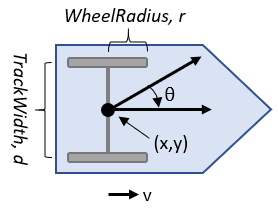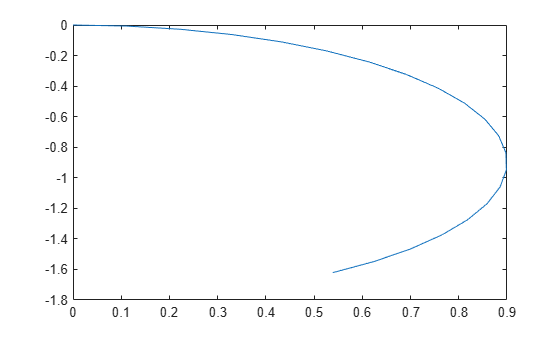# differentialDriveKinematics

Differential-drive vehicle model

## Description

`differentialDriveKinematics` creates a differential-drive vehicle model to simulate simplified vehicle dynamics. This model approximates a vehicle with a single fixed axle and wheels separated by a specified track width. The wheels can be driven independently. Vehicle speed and heading is defined from the axle center. The state of the vehicle is defined as a three-element vector, [x y theta], with a global xy-position, specified in meters, and a vehicle heading, theta, specified in radians. To compute the time derivative states for the model, use the `derivative` function with input commands and the current robot state.## Creation

### Syntax

``kinematicModel = differentialDriveKinematics``
``kinematicModel = differentialDriveKinematics(Name,Value)``

### Description

example

````kinematicModel = differentialDriveKinematics` creates a differential drive kinematic model object with default property values.```
````kinematicModel = differentialDriveKinematics(Name,Value)` sets properties on the object to the specified value. You can specify multiple properties in any order.```

## Properties

expand all

The wheel radius of the vehicle, specified in meters.

The vehicle speed range is a two-element vector that provides the minimum and maximum vehicle speeds, [MinSpeed MaxSpeed], specified in meters per second.

The vehicle track width refers to the distance between the wheels, or the axle length, specified in meters.

The `VehicleInputs` property specifies the format of the model input commands when using the `derivative` function. Options are specified as one of the following strings:

• `"WheelSpeeds"` — Angular speeds for each of the wheels, specified in radians per second.

• `"VehicleSpeedHeadingRate"` — Vehicle speed and heading angular velocity,specified in meters per second and radians per second respectively.

## Object Functions

 `derivative` Time derivative of vehicle state

## Examples

collapse all

Create a Robot

Define a robot and set the initial starting position and orientation.

```kinematicModel = differentialDriveKinematics; initialState = [0 0 0];```

Simulate Robot Motion

Set the timespan of the simulation to 1 s with 0.05 s timesteps and the input commands to 2 m/s and left turn. Simulate the motion of the robot by using the `ode45` solver on the `derivative` function.

```tspan = 0:0.05:1; inputs = [50 40]; %Left wheel is spinning faster [t,y] = ode45(@(t,y)derivative(kinematicModel,y,inputs),tspan,initialState);```

Plot Path

```figure plot(y(:,1),y(:,2))```Lynch, Kevin M., and Frank C. Park. Modern Robotics: Mechanics, Planning, and Control 1st ed. Cambridge, MA: Cambridge University Press, 2017.

##### Support평가판 신청